# SAT Math Multiple Choice Question 307: Answer and Explanation

### Test Information

Question: 307

7.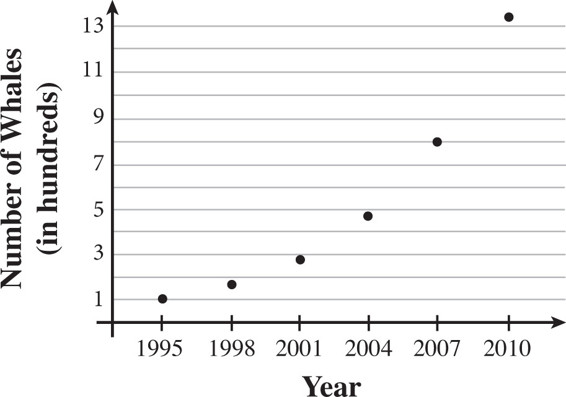The blue whale is the largest creature in the world and has been found in every ocean in the world. A marine biologist surveyed the blue whale population in Monterey Bay, off the coast of California, every three years between 1995 and 2010. The figure above shows her results. If w is the number of blue whales present in Monterey Bay and t is the number of years since the study began in 1995, which of the following equations best represents the blue whale population of Monterey Bay?

• A. w = 100 + 2t
• B.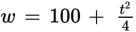• C.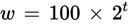• D.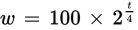Explanation:

D

Difficulty: Medium

Category: Passport to Advanced Math / Scatterplots

Strategic Advice: Use the shape of the data to predict the type of equation that might be used as a model. Then, use specific values from the graph to choose the correct equation.

Getting to the Answer: According to the graph, the population of the whales grew slowly at first and then more quickly. This means that an exponential model is probably the best fit, so you can eliminate A (linear) and B (quadratic). The remaining equations are both exponential, so choose a data point and see which equation is the closest fit. Be careful-the vertical axis represents hundreds of whales, and the question states that t represents the number of years since the study began, so t = 0 for 1995, t = 3 for 1998, and so on. If you use the data for 1995, which is the point (0, 100), the results are the same for both equations, so choose a different point. Using the data for 2007, t = 2007 - 1995 = 12, and the number of whales was 800. Substitute these values into C and D to see which one is true. Choice C is not true because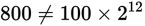. Choice (D) is correct because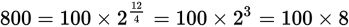is true.